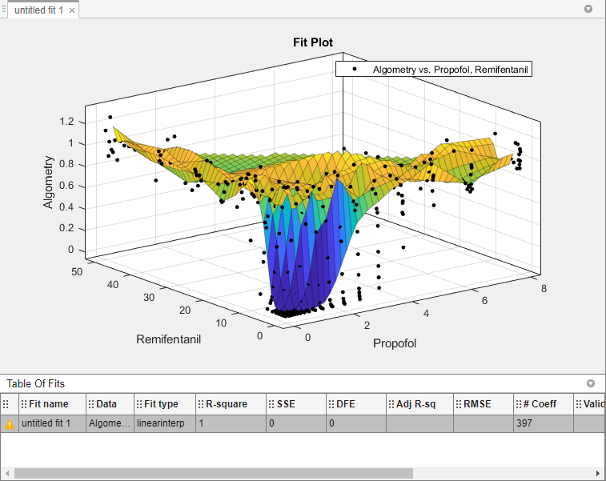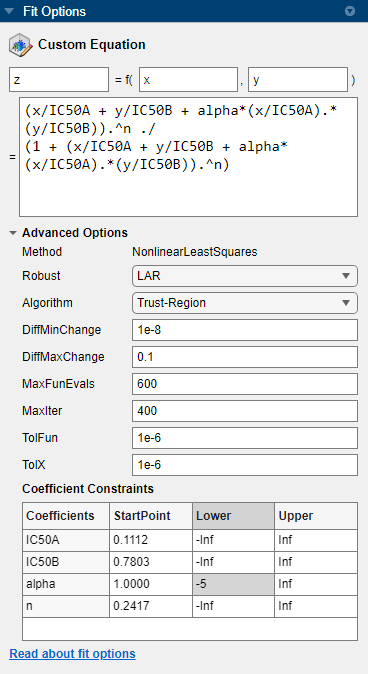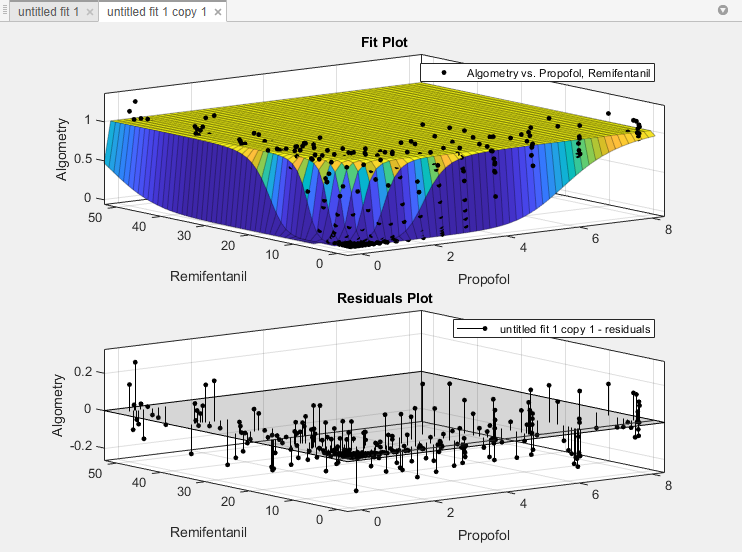## Surface Fitting to Biopharmaceutical Data

Curve Fitting Toolbox™ software provides some example data for an anesthesia drug interaction study. You can use the Curve Fitter app to fit response surfaces to this data to analyze drug interaction effects. Response surface models provide a good method for understanding the pharmacodynamic interaction behavior of drug combinations. This data is based on the results in .

Anesthesia is typically at least a two-drug process, consisting of an opioid and a sedative hypnotic. This example uses Propofol and Reminfentanil as drug class prototypes. Their interaction is measured by four different measures of the analgesic and sedative response to the drug combination. Algometry, Tetany, Sedation, and Laryingoscopy comprise the four measures of surrogate drug effects at various concentration combinations of Propofol and Reminfentanil.

To interactively create response surfaces for this drug combination:

1. Use the Current Folder browser to locate and view the folder `matlab\toolbox\curvefit\curvefit`.

2. Right-click the file `OpioidHypnoticSynergy.txt`, and select Import Data. The Import Tool appears.

1. On the Import tab, in the Delimiters section, leave the default Column delimiters value as `Tab`.

Review the six variables selected for import: `Propofol`, `Reminfentanil`, `Algometry`, `Tetany`, `Sedation`, and `Laryingoscopy`.

2. In the Import section, click and select Import Data to import the dose-response data into the MATLAB® workspace. Close the Import Tool.

Alternatively, you can import the data programmatically. Enter the following code to read the dose-response data from the file into the MATLAB workspace.

```data = importdata("OpioidHypnoticSynergy.txt"); OpioidHypnoticSynergy = array2table(data.data, ... "VariableNames",data.textdata);```
3. To create response surfaces, you must select two drugs as x and y inputs, and one of the four effects as the z output. After you load the variables into your workspace, you open the Curve Fitter app and then select variables interactively. Alternatively, you can specify the initial fit variables when using the `curveFitter` function.

For this example, open the Curve Fitter app.

`curveFitter`
4. In the Curve Fitter app, on the Curve Fitter tab, in the Data section, click . In the Select Fitting Data dialog box, first select the `OpioidHypnoticSynergy` table from the X data, Y data, and Z data drop-down lists. Then select the `Propofol`, `Remifentanil`, and `Algometry` variables from the new drop-down lists.The app creates a new response surface for the `Algometry` data. The default fit is an interpolating surface that passes through the data points.5. Create a copy of the current surface fit by either:

1. Selecting the button in the File section of the Curve Fitter tab.

2. Right-clicking a fit in the Table Of Fits pane, and selecting Duplicate "untitled fit 1".

6. Define your own equation to fit the data. On the Curve Fitter tab, in the Fit Type section, click the arrow to open the gallery. In the gallery, click in the Custom group.

7. In the Fit Options pane, select and delete the example custom equation text in the edit box.

You can use the custom equation edit box to enter MATLAB code to define your model. The equation that defines the model must depend on the data variables `x` and `y` and a list of fixed parameters, estimable parameters, or both.

The model from the paper is:

`$E=\frac{{E}_{\mathrm{max}}.{\left(\frac{{C}_{A}}{IC{50}_{A}}+\frac{{C}_{B}}{IC{50}_{B}}+\alpha .\frac{{C}_{A}}{IC{50}_{A}}.\frac{{C}_{B}}{IC{50}_{B}}\right)}^{n}}{1+{\left(\frac{{C}_{A}}{IC{50}_{A}}+\frac{{C}_{B}}{IC{50}_{B}}+\alpha .\frac{{C}_{A}}{IC{50}_{A}}.\frac{{C}_{B}}{IC{50}_{B}}\right)}^{n}}$`

where CA and CB are the drug concentrations, and IC50A, IC50B, alpha, and n are the coefficients to be estimated.

You can define this in MATLAB code as:

```Effect = Emax*(CA/IC50A + CB/IC50B + ... alpha*(CA/IC50A).*(CB/IC50B)).^n ./ ... (1 + (CA/IC50A + CB/IC50B + ... alpha*(CA/IC50A ).*(CB/IC50B)).^n);```

Telling the app which variables to fit and which parameters to estimate requires rewriting the variable names `CA` and `CB` as `x`, and `y`. You must include `x` and `y` when you enter a custom equation in the edit box. Assume `Emax = 1` because the effect output is normalized.

8. Enter the following text in the custom equation edit box.

```(x/IC50A + y/IC50B + alpha*(x/IC50A).*(y/IC50B)).^n ./ (1 + (x/IC50A + y/IC50B + alpha*(x/IC50A).*(y/IC50B)).^n)```

The Curve Fitter app fits a surface to the data using the custom equation model.9. In the Fit Options pane, change some of the fit options. Click Advanced Options to expand the section.

1. Set the Robust value to `LAR`.

2. In the Coefficient Constraints table, for the alpha coefficient, set the StartPoint value to `1` and the Lower bound to `–5`.10. Review the Results pane. View any of these results:

• The model equation

• The values of the estimated coefficients

• The goodness-of-fit statistics

11. Display the residuals plot to check the distribution of points relative to the surface. On the Curve Fitter tab, in the Visualization section, click .12. Generate code for the currently selected fit and its open plots in your Curve Fitter app session. On the Curve Fitter tab, in the Export section, click and select Generate Code.

The Curve Fitter app generates code from your session and displays the file in the MATLAB Editor. The file includes the fit selected in your current session and its open plots.

13. Save the file with the default name, `createFit.m`.

14. You can recreate your fit and its plots by calling the file from the command line with your original data or new data as input arguments.

In this case, because your original data still appears in the workspace, you can run the function with the original data variables.

```[fitresult,gof] = createFit(OpioidHypnoticSynergy.Propofol, ... OpioidHypnoticSynergy.Remifentanil, ... OpioidHypnoticSynergy.Algometry)```

The function creates a figure window for the fit you selected in your session. The custom fit figure shows both the surface and residuals plots that you created interactively in the Curve Fitter app.

15. Create a new fit to the `Tetany` response instead of `Algometry`.

```[fitresult,gof] = createFit(OpioidHypnoticSynergy.Propofol, ... OpioidHypnoticSynergy.Remifentanil, ... OpioidHypnoticSynergy.Tetany)```

You need to edit the file if you want the new response label on the plots. You can use the generated code as a starting point to change the surface fits and plots to meet your needs. For a list of methods you can use, see `sfit`.

To see how to programmatically fit surfaces to the same example problem, see Surface Fitting with Custom Equations to Biopharmaceutical Data.

 Kern, Steven E., Guoming Xie, Julia L. White, and Talmage D. Egan. “A Response Surface Analysis of Propofol–Remifentanil Pharmacodynamic Interaction in Volunteers.” Anesthesiology 100, no. 6 (June 1, 2004): 1373–81. https://doi.org/10.1097/00000542-200406000-00007.